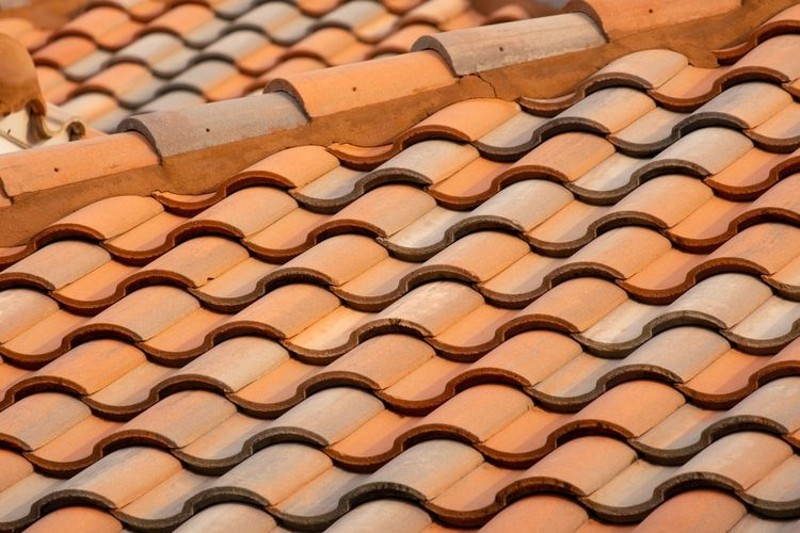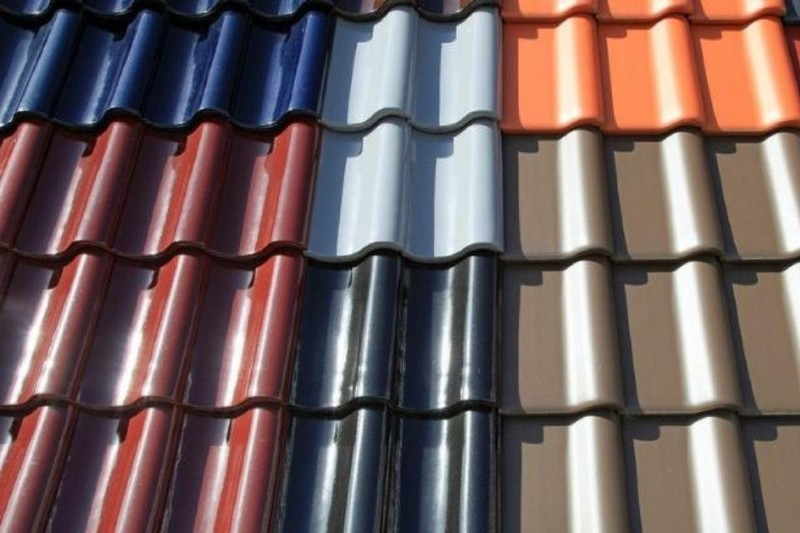Breaking News# How to Calculate the Need for Roof Roofing Based on the Formula

How to Calculate the Need for Roof Roofing Based on the FormulaDo you want to install tiles on the roof of the house, but confused about how to calculate the tile requirements? Don’t worry, try using the following two formulas, let’s go!

Having a function to protect the house and its occupants from heat, pollution, rain, installing a tile roof on a house is important to do.

Even so, the installation of tiles should not be done without care. You need the right calculation so as not to lose.

Do not leave the purchase of tiles much above or even less than the need.

The reason is, if the amount of tiles purchased exceeds the need, it will be redundant.

But if the number is less, you will certainly be confused to find the same type of tile.

So you can know the exact number of tiles needed. Follow how to calculate the tile requirements with the formula below, go!

## The formula to calculate the need for tiles for the roof of the houseSource: Kompas.com

### 1. How to Calculate Tile Needed by Roof Area

The easiest way to calculate the tile needs is based on the area of ​​the roof.

See also  Understand the dangers of household waste and how to deal with it

For example, the construction of a house with a roof area calculation of 10 x 9 m = 90 m2.

Then, the result is multiplied by 2 sides of the roof. Therefore, 90 x 2 = 180 m2

This total can be a reference when you buy a house tile.

For example, for 1 m2 of roof contains 25 pieces, then the calculation is:

180 m2 x 25 = 4,500 tiles.

This figure can be said to be accurate. In any case, it is highly recommended to slightly increase the number of tiles that will be purchased in advance if the tiles are damaged.

### 2. How to Calculate Tile Needs Based on the Length and Width of the Foundation

The next method can be calculated by looking at the length and width of the foundation of the house.

However, if you use this method, it is better to add about 1 m more to the length and width.

If you are still confused, try to see the example below.

A house is 9 m long and 7 m wide. If you add 1 m to the length and width, the calculation is as follows:

10 x 8 m = 80 m2.

Then, multiply the area by 35 so that it becomes 80 m2 x 35 = 2,800 tiles.

However, it should be noted that this calculation only covers the core part of the house.

If there are other parts, such as terraces or balconies, how to calculate the need for tiles on the above formula can be repeated.

## Several tips for purchasing quality home tilesSource: Mamikos.com

The quality tile can protect the house well. The following are some tips for buying good quality home tiles.

### 1. Customize with Budget and Needs

adapt budget it sounds like a trivial matter. However, this is crucial, that’s it.

Do not let the production exceed the set budget.

To adapt to budgetchoose the type of tile according to the needs of the house.

### 2. Adjust to the Strength of the House Frame

Each type of tile has different characteristics.

The easiest way to choose the type of tile according to your needs is to choose the type of tile based on the strength of the frame of the house.

For example, the frame of the house has standard strength. Thus, you can consider the type of tile with a light weight.

### 3. Check Condition Tile

When choosing a tile, pay attention to the condition of the surface. Avoid buying tiles that have cracks, even if it’s small.

Because, it can affect the function of the tile itself.

Try to choose a tile with a smooth surface.

### 4. Do a Leak Check

If allowed by the seller, you should also check for leaks on the tile.

The trick, you can sprinkle water on the surface.

### 5. Tap the Tile to check the quality

To ensure the quality of the tile, you can also try to touch the surface.

Even so, this method does not apply to all tiles. In a sense, it can only be done for certain types of tiles.

The tiles that you can check with this method are tiles made of clay.

Instead, choose a tile that when touched does not make a loud sound.

See also  11 good and fair ways to get rid of cats without injury

Well, this is a variety of ways to easily calculate the need for tiles.